Home
Hostname: page-component-558cb97cc8-4xlcd Total loading time: 0.854 Render date: 2022-10-06T10:07:19.536Z Has data issue: true Feature Flags: { "shouldUseShareProductTool": true, "shouldUseHypothesis": true, "isUnsiloEnabled": true, "useRatesEcommerce": false, "displayNetworkTab": true, "displayNetworkMapGraph": true, "useSa": true } hasContentIssue true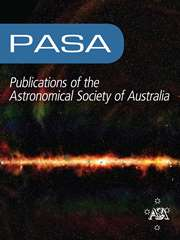Publications of the Astronomical Society of Australia

# The formation of planetary systems with SPICA

Published online by Cambridge University Press:  03 November 2021

*
*Author for correspondence: Inga Kamp, E-mail: kamp@astro.rug.nl

## Abstract

In this era of spatially resolved observations of planet-forming disks with Atacama Large Millimeter Array (ALMA) and large ground-based telescopes such as the Very Large Telescope (VLT), Keck, and Subaru, we still lack statistically relevant information on the quantity and composition of the material that is building the planets, such as the total disk gas mass, the ice content of dust, and the state of water in planetesimals. SPace Infrared telescope for Cosmology and Astrophysics (SPICA) is an infrared space mission concept developed jointly by Japan Aerospace Exploration Agency (JAXA) and European Space Agency (ESA) to address these questions. The key unique capabilities of SPICA that enable this research are (1) the wide spectral coverage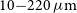$10{-}220\,\mu\mathrm{m}$ , (2) the high line detection sensitivity of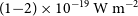$(1{-}2) \times 10^{-19}\,\mathrm{W\,m}^{-2}$ with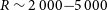$R \sim 2\,000{-}5\,000$ in the far-IR (SAFARI), and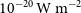$10^{-20}\,\mathrm{W\,m}^{-2}$ with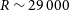$R \sim 29\,000$ in the mid-IR (SPICA Mid-infrared Instrument (SMI), spectrally resolving line profiles), (3) the high far-IR continuum sensitivity of 0.45 mJy (SAFARI), and (4) the observing efficiency for point source surveys. This paper details how mid- to far-IR infrared spectra will be unique in measuring the gas masses and water/ice content of disks and how these quantities evolve during the planet-forming period. These observations will clarify the crucial transition when disks exhaust their primordial gas and further planet formation requires secondary gas produced from planetesimals. The high spectral resolution mid-IR is also unique for determining the location of the snowline dividing the rocky and icy mass reservoirs within the disk and how the divide evolves during the build-up of planetary systems. Infrared spectroscopy (mid- to far-IR) of key solid-state bands is crucial for assessing whether extensive radial mixing, which is part of our Solar System history, is a general process occurring in most planetary systems and whether extrasolar planetesimals are similar to our Solar System comets/asteroids. We demonstrate that the SPICA mission concept would allow us to achieve the above ambitious science goals through large surveys of several hundred disks within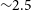$\sim\!2.5$ months of observing time.

Type
Research Article
© The Author(s), 2021. Published by Cambridge University Press on behalf of the Astronomical Society of Australia

## Access options

Get access to the full version of this content by using one of the access options below. (Log in options will check for institutional or personal access. Content may require purchase if you do not have access.)

## References

Partnership, ALMA, et al. 2015, ApJ, 808, L3 10.1088/2041-8205/803/1/L3CrossRefGoogle Scholar
Aikawa, Y., et al. 2012, A&A, 538, A57 10.1051/0004-6361/201015999CrossRefGoogle Scholar
Alexander, R., Pascucci, I., Andrews, S., Armitage, P., & Cieza, L. 2014, in Beuther, H., Klessen, R. S., Dullemond, C. P., & Henning, T., eds, Protostars and Planets VI. p. 475 (arXiv:1311.1819), doi: 10.2458/azu_uapress_9780816531240-ch021 CrossRefGoogle Scholar
Alexander, C. M. O., McKeegan, K. D., & Altwegg, K. 2018, Space Sci. Rev., 214, 3610.1007/s11214-018-0474-9CrossRefGoogle Scholar
Andrews, S. M., Rosenfeld, K. A., Kraus, A. L., & Wilner, D. J., 2013, ApJ, 771, 12910.1088/0004-637X/771/2/129CrossRefGoogle Scholar
Andrews, S. M., et al. 2018, ApJ, 869, L41 10.3847/2041-8213/aaf741CrossRefGoogle Scholar
Ansdell, M., et al. 2016, ApJ, 828, 4610.3847/0004-637X/828/1/46CrossRefGoogle Scholar
Ansdell, M., et al. 2018, ApJ, 859, 21 CrossRefGoogle Scholar
Antonellini, S. 2016, PhD thesis, Kapteyn Astronomical Institute, University of Groningen, Landleven 12, 9700 AV Groningen, The Netherlands E:Mail: Google Scholar
Antonellini, S., et al. 2015, A&A, 582, A105 10.1051/0004-6361/201525724CrossRefGoogle Scholar
Antonellini, S., et al. 2016, A&A, 585, A61 CrossRefGoogle Scholar
Baldovin-Saavedra, C., Audard, M., Carmona, A., Güdel, M., Briggs, K., Rebull, L. M., Skinner, S. L., & Ercolano, B. 2012, A&A, 543, A30 10.1051/0004-6361/201118329CrossRefGoogle Scholar
Ballering, N. P., & Eisner, J. A., 2019, AJ, 157, 14410.3847/1538-3881/ab0a56CrossRefGoogle Scholar
Banzatti, A., Pontoppidan, K. M., Salyk, C., Herczeg, G. J., van Dishoeck, E. F., & Blake, G. A. 2017, ApJ, 834, 152 10.3847/1538-4357/834/2/152CrossRefGoogle Scholar
Banzatti, A., Pascucci, I., Edwards, S., Fang, M., Gorti, U., & Flock, M. 2019, ApJ, 870, 76 CrossRefGoogle Scholar
Barenfeld, S. A., Carpenter, J. M., Ricci, L., & Isella, A. 2016, ApJ, 827, 142 10.3847/0004-637X/827/2/142CrossRefGoogle Scholar
Baruteau, C., et al. 2014, in Beuther, H., Klessen, R. S., Dullemond, C. P., & Henning, T., eds, Protostars and Planets VI. p. 667 (arXiv:1312.4293), doi: 10.2458/azu_uapress_9780816531240-ch029 CrossRefGoogle Scholar
Bary, J. S., Weintraub, D. A., & Kastner, J. H. 2003, ApJ, 586, 1136 10.1086/367719CrossRefGoogle Scholar
Beck, T. L., Bary, J. S., Dutrey, A., Piétu, V., Guilloteau, S., Lubow, S. H., & Simon, M. 2012, ApJ, 754, 72 CrossRefGoogle Scholar
Beck, P., Garenne, A., Quirico, E., Bonal, L., Montes-Hernandez, G., Moynier, F., & Schmitt, B. 2014, ICARUS, 229, 263 10.1016/j.icarus.2013.10.019CrossRefGoogle Scholar
Beckwith, S. V. W., Sargent, A. I., Chini, R. S., & Guesten, R. 1990, AJ, 99, 924 CrossRefGoogle Scholar
Benisty, M., et al. 2015, A&A, 578, L6 Google Scholar
Bergin, E. A., et al. 2013, Nature, 493, 644 10.1038/nature11805CrossRefGoogle Scholar
Birnstiel, T., Fang, M., & Johansen, A. 2016, Space Sci. Rev., 205, 4110.1007/s11214-016-0256-1CrossRefGoogle Scholar
Bockelée-Morvan, D., & Biver, N. 2017, PTRSL, 375, 20160252 10.1098/rsta.2016.0252CrossRefGoogle Scholar
Booth, A. S., & Ilee, J. D. 2020, MNRAS, 493, L108 10.1093/mnrasl/slaa014CrossRefGoogle Scholar
Booth, A. S., Walsh, C., Ilee, J. D., Notsu, S., Qi, C., Nomura, H., & Akiyama, E. 2019, ApJ, 882, L31 CrossRefGoogle Scholar
Bowey, J. E., et al. 2002, MNRAS, 331, L1 10.1046/j.1365-8711.2002.05349.xCrossRefGoogle Scholar
Brownlee, D. 2014, Annual Review of Earth and Planetary Sciences, 42, 179 CrossRefGoogle Scholar
Bruderer, S. 2013, A&A, 559, A46 CrossRefGoogle Scholar
Bruderer, S., van Dishoeck, E. F., Doty, S. D., & Herczeg, G. J. 2012, A&A, 541, A91 10.1051/0004-6361/201118218CrossRefGoogle Scholar
Calmonte, U., et al. 2016, MNRAS, 462, S253 10.1093/mnras/stw2601CrossRefGoogle Scholar
Carr, J. S., & Najita, J. R., 2008, Science, 319, 1504 10.1126/science.1153807CrossRefGoogle Scholar
Carr, J. S., Tokunaga, A. T., & Najita, J., 2004, ApJ, 603, 213 CrossRefGoogle Scholar
Carr, J. S., Najita, J. R., & Salyk, C. 2018, RNAAS, 2, 169 CrossRefGoogle Scholar
Casassus, S., et al. 2013, Nature, 493, 191 CrossRefGoogle Scholar
Cataldi, G., et al. 2018, ApJ, 861, 72 10.3847/1538-4357/aac5f3CrossRefGoogle Scholar
Cataldi, G., et al. 2020, ApJ, 892, 99 10.3847/1538-4357/ab7cc7CrossRefGoogle Scholar
Cazzoletti, P., et al. 2019, A&A, 626, A11 Google Scholar
Ceccarelli, C., Caux, E., Tielens, A. G. G. M., Kemper, F., Waters, L. B. F. M., & Phillips, T. 2002, A&A, 395, L29 CrossRefGoogle Scholar
Chyba, C. F., Thomas, P. J., Brookshaw, L., & Sagan, C. 1990, Science, 249, 366 CrossRefGoogle Scholar
Cieza, L. A., et al. 2019, MNRAS, 482, 698 Google Scholar
Cleeves, L. I., Öberg, K. I., Wilner, D. J., Huang, J., Loomis, R. A., Andrews, S. M., & Guzman, V. V. 2018, ApJ, 865, 155 10.3847/1538-4357/aade96CrossRefGoogle Scholar
Cotten, T. H., & Song, I. 2016, in Kastner, J. H., Stelzer, B., & Metchev, S. A., eds, IAU Symposium Vol. 314, Young Stars & Planets Near the Sun, pp 179–182 (arXiv:1510.01142), doi: 10.1017/S1743921315006389 CrossRefGoogle Scholar
Crovisier, J., Leech, K., Bockelee-Morvan, D., Brooke, T. Y., Hanner, M. S., Altieri, B., Keller, H. U., & Lellouch, E. 1997, Science, 275, 1904 CrossRefGoogle Scholar
D’Angelo, M., Cazaux, S., Kamp, I., Thi, W. F., Woitke, P. 2019, A&A, 622, A208 10.1051/0004-6361/201833715CrossRefGoogle Scholar
Dartois, E., Dutrey, A., & Guilloteau, S. 2003, A&A, 399, 773 10.1051/0004-6361:20021638CrossRefGoogle Scholar
Dent, W. R. F., et al. 2013, PASP, 125, 477 Google Scholar
Dent, W. R. F., et al. 2014, Science, 343, 1490 CrossRefGoogle Scholar
Dipierro, G., Pinilla, P., Lodato, G., & Testi, L. 2015, MNRAS, 451, 974 CrossRefGoogle Scholar
Eistrup, C., Walsh, C., & van Dishoeck, E. F. 2016, A&A, 595, A83 CrossRefGoogle Scholar
Ercolano, B., & Pascucci, I. 2017, Royal Society Open Science, 4, 170114 10.1098/rsos.170114CrossRefGoogle Scholar
Evans Neal, J. I., et al. 2009, ApJs, 181, 321 10.1016/j.juro.2008.09.141CrossRefGoogle Scholar
Fedele, D., van den Ancker, M. E., Henning, T., Jayawardhana, R., & Oliveira, J. M. 2010, A&A, 510, A72 CrossRefGoogle Scholar
Fedele, D., Bruderer, S., van Dishoeck, E. F., Herczeg, G. J., Evans, N. J., Bouwman, J., Henning, T., & Green, J. 2012, A&A, 544, L9 CrossRefGoogle Scholar
Fedele, D., van Dishoeck, E. F., Kama, M., Bruderer, S., & Hogerheijde, M. R. 2016, A&A, 591, A95 10.1051/0004-6361/201526948CrossRefGoogle Scholar
Flower, D. R., & Pineau des Forêts, G., 2015, A&A, 578, A63 10.1051/0004-6361/201525740CrossRefGoogle Scholar
Font, A. S., McCarthy, I. G., Johnstone, D., & Ballantyne, D. R. 2004, ApJ, 607, 890 CrossRefGoogle Scholar
Gail, H. P. 2004, A&A, 413, 571 10.1051/0004-6361:20031554CrossRefGoogle Scholar
Ginski, C., et al. 2018, A&A, 616, A79 CrossRefGoogle Scholar
Glassgold, A. E., Meijerink, R., & Najita, J. R. 2009, ApJ, 701, 142 10.1088/0004-637X/701/1/142CrossRefGoogle Scholar
Haisch Karl, E. J., Lada, E. A., Lada, C. J. 2001, ApJ, 553, L153 10.1086/320685CrossRefGoogle Scholar
Hamilton, V. E., et al. 2019, NatAs, 3, 332 Google Scholar
Hardy, A., et al. 2015, A&A, 583, A66 10.1051/0004-6361/201526504CrossRefGoogle Scholar
Hartigan, P., Edwards, S., & Ghandour, L. 1995, ApJ, 452, 736 CrossRefGoogle Scholar
Hayashi, C. 1981, Prog. Theoretical Phys. Suppl., 70, 35 CrossRefGoogle Scholar
Heays, A. N., Bosman, A. D., & van Dishoeck, E. F., 2017, A&A, 602, A105 10.1051/0004-6361/201628742CrossRefGoogle Scholar
Helling, C., Woitke, P., Rimmer, P. B., Kamp, I., Thi, W.-F., & Meijerink, R. 2014, Life, 4, 142 CrossRefGoogle Scholar
Hernández, J., Hartmann, L., Calvet, N., Jeffries, R. D., Gutermuth, R., Muzerolle, J., & Stauffer, J. 2008, ApJ, 686, 1195 CrossRefGoogle Scholar
Hogerheijde, M. R., et al. 2011, Science, 334, 338 CrossRefGoogle Scholar
Honda, M., et al. 2009, ApJ, 690, L110 CrossRefGoogle Scholar
Honda, M., et al. 2016, ApJ, 821, 2 CrossRefGoogle Scholar
Huang, J., et al. 2018, ApJ, 869, L42 CrossRefGoogle Scholar
Juhász, A., et al. 2010, ApJ, 721, 431 CrossRefGoogle Scholar
Kama, M., et al. 2020, A&A, 634, A88 CrossRefGoogle Scholar
Kamp, I., Woitke, P., Pinte, C., Tilling, I., Thi, W. F., Menard, F., Duchene, G., & Augereau, J. C. 2011, A&A, 532, A85 10.1051/0004-6361/201016399CrossRefGoogle Scholar
Kamp, I., et al. 2013, A&A, 559, A24 CrossRefGoogle Scholar
Kamp, I., Thi, W.-F., Woitke, P., Rab, C., Bouma, S., & Ménard, F. 2017, A&A, 607, A41 CrossRefGoogle Scholar
Kamp, I., Antonellini, S., Carmona, A., Ilee, J., & Rab, C., 2018, in Ootsubo, T., Yamamura, I., Murata, K., & Onaka, T., eds, The Cosmic Wheel and the Legacy of the AKARI Archive: From Galaxies and Stars to Planets and Life. pp 89–96 (arXiv:1712.00303)Google Scholar
Kemper, F., Jäger, C., Waters, L. B. F. M., Henning, T., Molster, F. J., Barlow, M. J., Lim, T., & de Koter, A. 2002, Nature, 415, 295 CrossRefGoogle Scholar
Keppler, M., et al. 2018, A&A, 617, A44 Google Scholar
Kikuchi, A., Higuchi, A., & Ida, S. 2014, ApJ, 797, 1 10.1088/0004-637X/797/1/1CrossRefGoogle Scholar
Kitazato, K., et al. 2019, Science, 364, 272 Google Scholar
Koike, C., & Shibai, H. 1990, MNRAS, 246, 332 Google Scholar
Kral, Q., 2016, in SF2A-2016: Proceedings of the Annual meeting of the French Society of Astronomy and Astrophysics. pp 463–472 (arXiv:1611.06751)Google Scholar
Kral, Q., & Latter, H. 2016, MNRAS, 461, 1614 10.1093/mnras/stw1429CrossRefGoogle Scholar
Kral, Q., Wyatt, M., Carswell, R. F., Pringle, J. E., Matrà, L., & Juhász, A., 2016, MNRAS, 461, 845 CrossRefGoogle Scholar
Kral, Q., Matrà, L., Wyatt, M. C., & Kennedy, G. M. 2017, MNRAS, 469, 521 CrossRefGoogle Scholar
Kral, Q., Wyatt, M. C., Triaud, A. H. M. J., Marino, S., Thébault, P., & Shorttle, O. 2018, MNRAS, 479, 2649 CrossRefGoogle Scholar
Kral, Q., Marino, S.,Wyatt, M. C., Kama, M., & Matrà, L. 2019, MNRAS, 489, 3670 Google Scholar
Kral, Q., Davoult, J., & Charnay, B. 2020a, Nature Astronomy, 4, 769 CrossRefGoogle Scholar
Kral, Q., Matrà, L., Kennedy, G. M., Marino, S., & Wyatt, M. C. 2020b, MNRAS, 497, 2811 10.1093/mnras/staa2038CrossRefGoogle Scholar
Lahuis, F., van Dishoeck, E. F., Blake, G. A., Evans Neal, J. I., Kessler-Silacci, J. E., & Pontoppidan, K. M. 2007, ApJ, 665, 492 CrossRefGoogle Scholar
Lammer, H., & Blanc, M. 2018, Space Sci. Rev., 214, 60 CrossRefGoogle Scholar
Lammer, H., et al. 2014, MNRAS, 439, 3225 CrossRefGoogle Scholar
Larimer, J. W. 1975, Geochim. Cosmochim. Acta, 39, 389 CrossRefGoogle Scholar
Lissauer, J. J., & Stevenson, D. J. 2007, in Reipurth, B., Jewitt, D., & Keil, K., eds, Protostars and Planets V. p. 591Google Scholar
Long, F., et al. 2018, ApJ, 869, 17 Google Scholar
Long, F., et al. 2019, ApJ, 882, 49 CrossRefGoogle Scholar
Lovell, J. B., et al. 2020, MNRAS, 500, 48784900 CrossRefGoogle Scholar
Maaskant, K. M., de Vries, B. L., Min, M., Waters, L. B. F. M., Dominik, C., Molster, F., & Tielens, A. G. G. M. 2015, A&A, 574, A140 CrossRefGoogle Scholar
Malfait, K., Waelkens, C., Waters, L. B. F. M., Vandenbussche, B., Huygen, E., de Graauw, M. S. 1998, A&A, 332, L25 Google Scholar
Malfait, K., Waelkens, C., Bouwman, J., de Koter, A., & Waters, L. B. F. M. 1999, A&A, 345, 181 Google Scholar
Mamajek, E. E. 2009, in Usuda, T., Tamura, M., & Ishii, M., eds, American Institute of Physics Conference Series Vol. 1158, American Institute of Physics Conference Series. pp. 3–10 (arXiv:0906.5011), doi: 10.1063/1.3215910 CrossRefGoogle Scholar
Marino, S., et al. 2016, MNRAS, 460, 2933 CrossRefGoogle Scholar
Massol, H., et al. 2016, Space Sci. Rev., 205, 153 CrossRefGoogle Scholar
Matrà, L., Panić, O., Wyatt, M. C., Dent, & W. R. F. 2015, MNRAS, 447, 3936 CrossRefGoogle Scholar
Matrà, L., et al. 2017, ApJ, 842, 9 CrossRefGoogle Scholar
Matrà, L., Wilner, D. J., Öberg, K. I., Andrews, S. M., Loomis, R. A., Wyatt, M. C., & Dent, W. R. F. 2018, ApJ, 853, 147 10.3847/1538-4357/aaa42aCrossRefGoogle Scholar
Matrà, L., Wyatt, M. C., Wilner, D. J., Dent, W. R. F., Marino, S., Kennedy, G. M., & Milli, J. 2019, AJ, 157, 135 10.3847/1538-3881/ab06c0CrossRefGoogle Scholar
McClure, M. K., et al. 2012, ApJ, 759, L10 CrossRefGoogle Scholar
McClure, M. K., et al. 2015, ApJ, 799, 162 CrossRefGoogle Scholar
McClure, M. K., et al. 2016, ApJ, 831, 167 CrossRefGoogle Scholar
Meeus, G., et al. 2012, A&A, 544, A78 CrossRefGoogle Scholar
Min, M., Hovenier, J. W., de Koter, A. 2005, A&A, 432, 909 CrossRefGoogle Scholar
Min, M., Rab, C., Woitke, P., Dominik, C., & Ménard, F. 2016a, A&A, 585, A13 CrossRefGoogle Scholar
Min, M., et al. 2016b, A&A, 593, A11 Google Scholar
Miotello, A., Bruderer, S., & van Dishoeck, E. F. 2014, A&A, 572, A96 CrossRefGoogle Scholar
Miotello, A., van Dishoeck, E. F., Kama, M., & Bruderer, S. 2016, A&A, 594, A85 CrossRefGoogle Scholar
Miotello, A., et al. 2017, A&A, 599, A113 CrossRefGoogle Scholar
Miotello, A., et al. 2019, A&A, 631, A69 CrossRefGoogle Scholar
Molyarova, T., Akimkin, V., Semenov, D., Henning, T., Vasyunin, A., & Wiebe, D. 2017, ApJ, 849, 130 CrossRefGoogle Scholar
Moór, A., et al. 2019, ApJ, 884, 108 CrossRefGoogle Scholar
Moriarty, J., Madhusudhan, N., & Fischer, D. 2014, ApJ, 787, 81 CrossRefGoogle Scholar
Muto, T., Takeuchi, T., & Ida, S. 2011, ApJ, 737, 37 CrossRefGoogle Scholar
Natta, A., Testi, L., Alcalá, J. M., Rigliaco, E., Covino, E., Stelzer, B., & D’Elia, V. 2014, A&A, 569, A5 CrossRefGoogle Scholar
Nisini, B., Antoniucci, S., Alcalá, J. M., Giannini, T., Manara, C. F., Natta, A., Fedele, D., & Biazzo, K. 2018, A&A, 609, A87 CrossRefGoogle Scholar
Nittler, L. R., & Ciesla, F. 2016, ARA&A, 54, 53 CrossRefGoogle Scholar
Nomura, H., Aikawa, Y., Tsujimoto, M., Nakagawa, Y., & Millar, T. J., 2007, ApJ, 661, 334 CrossRefGoogle Scholar
Notsu, S., Nomura, H., Ishimoto, D., Walsh, C., Honda, M., Hirota, T., & Millar, T. J. 2016, ApJ, 827, 113 CrossRefGoogle Scholar
Notsu, S., Nomura, H., Ishimoto, D., Walsh, C., Honda, M., Hirota, T., & Millar, T. J. 2017, ApJ, 836, 118 CrossRefGoogle Scholar
Notsu, S., Nomura, H., Walsh, C., Honda, M., Hirota, T., Akiyama, E., & Millar, T. J. 2018, ApJ, 855, 62 CrossRefGoogle Scholar
Notsu, S., et al. 2019, ApJ, 875, 96 CrossRefGoogle Scholar
Notsu, S., Eistrup, C., Walsh, C., Nomura, H. 2020, MNRAS, 499, 2229 CrossRefGoogle Scholar
Öberg, K. I., Murray-Clay, R., & Bergin, E. A. 2011, ApJ, 743, L16 CrossRefGoogle Scholar
Ohsawa, R., Onaka, T., & Yasui, C., 2015, PASJ, 67, 120 CrossRefGoogle Scholar
Okuzumi, S., Tanaka, H., Kobayashi, H., & Wada, K. 2012, ApJ, 752, 106 CrossRefGoogle Scholar
Paardekooper, S. J., & Mellema, G. 2006, A&A, 453, 1129 CrossRefGoogle Scholar
Panić, O., & Hogerheijde, M. R. 2009, A&A, 508, 707 CrossRefGoogle Scholar
Pascucci, I., et al. 2007, ApJ, 663, 383 CrossRefGoogle Scholar
Pascucci, I., et al. 2011, ApJ, 736, 13 CrossRefGoogle Scholar
Pascucci, I., et al. 2016, ApJ, 831, 125 CrossRefGoogle Scholar
Pérez, L. M., Isella, A., Carpenter, J. M., & Chandler, C. J. 2014, ApJ, 783, L13 CrossRefGoogle Scholar
Picogna, G., Ercolano, B., Owen, J. E., & Weber, M. L. 2019, MNRAS, 487, 691 CrossRefGoogle Scholar
Pinte, C., et al. 2018, ApJ, 860, L13 CrossRefGoogle Scholar
Pontoppidan, K. M., Dullemond, C. P., van Dishoeck, E. F., Blake, G. A., Boogert, A. C. A., Evans Neal, J. I., Kessler-Silacci, J. E., & Lahuis, F. 2005, ApJ, 622, 463 CrossRefGoogle Scholar
Pontoppidan, K. M., Salyk, C., Blake, G. A., Meijerink, R., Carr, J. S., & Najita, J. 2010, ApJ, 720, 887 CrossRefGoogle Scholar
Prodanović, T., Steigman, G., & Fields, B. D. 2010, MNRAS, 406, 1108 Google Scholar
Rapson, V. A., Kastner, J. H., Andrews, S. M., Hines, D. C., Macintosh, B., Millar-Blanchaer, M., & Tamura, M. 2015, ApJ, 803, L10 CrossRefGoogle Scholar
Richert, A. J. W., Getman, K. V., Feigelson, E. D., Kuhn, M. A., Broos, P. S., Povich, M. S., Bate, M. R., & Garmire, G. P. 2018, MNRAS, 477, 5191 CrossRefGoogle Scholar
Rigliaco, E., Pascucci, I., Gorti, U., Edwards, S., & Hollenbach, D., 2013, ApJ, 772, 60 10.1088/0004-637X/772/1/60CrossRefGoogle Scholar
Riviere-Marichalar, P., et al. 2012, A&A, 538, L3 CrossRefGoogle Scholar
Riviere-Marichalar, P., et al. 2014, A&A, 565, A68 CrossRefGoogle Scholar
Riviere-Marichalar, P., Merín, B., Kamp, I., Eiroa, C., & Montesinos, B. 2016, A&A, 594, A59 CrossRefGoogle Scholar
Roberge, A., Feldman, P. D.,Weinberger, A. J., Deleuil, M., & Bouret, J.-C. 2006, Nature, 441, 724 CrossRefGoogle Scholar
Rocha, W. R. M., & Pilling, S. 2020, ApJ, 896, 27 CrossRefGoogle Scholar
Roelfsema, P. R., et al. 2018, PASA, 35, e030 Google Scholar
Salyk, C., Pontoppidan, K. M., Blake, G. A., Najita, J. R., & Carr, J. S. 2011a, ApJ, 731, 130 CrossRefGoogle Scholar
Salyk, C., Blake, G. A., Boogert, A. C. A., & Brown, J. M. 2011b, ApJ, 743, 112 CrossRefGoogle Scholar
Sargent, B. A., et al. 2009, ApJS, 182, 477 CrossRefGoogle Scholar
Schoonenberg, D., & Ormel, C. W. 2017, A&A, 602, A21 CrossRefGoogle Scholar
Shu, F. H., Johnstone, D., & Hollenbach, D. 1993, ICARUS, 106, 92 10.1006/icar.1993.1160CrossRefGoogle Scholar
Simon, M. N., Pascucci, I., Edwards, S., Feng, W., Gorti, U., Hollenbach, D., Rigliaco, E., & Keane, J. T. 2016, ApJ, 831, 169 CrossRefGoogle Scholar
Smith, R. G., Robinson, G., Hyland, A. R., & Carpenter, G. L. 1994, Mon. Not. R. Astron. Soc., 271, 481 CrossRefGoogle Scholar
Sturm, B., et al. 2010, A&A, 518, L129 Google Scholar
Sturm, B., et al. 2013, A&A, 553, 5 Google Scholar
Suto, H., et al. 2006, MNRAS, 370, 1599 CrossRefGoogle Scholar
Tanaka, H., & Ward, W. R., 2004, ApJ, 602, 388 CrossRefGoogle Scholar
Terada, H., & Tokunaga, A. T. 2017, ApJ, 834, 115 CrossRefGoogle Scholar
Thi, W. F., Hocuk, S., Kamp, I., Woitke, P., Rab, C., Cazaux, S., Caselli, P., & D’Angelo, M. 2020, A&A, 635, A16 CrossRefGoogle Scholar
Toppani, A., Robert, F., Libourel, G., de Donato, P., Barres, O., D’Hendecourt, L., & Ghanbaja, J. 2005, Nature, 437, 1121 10.1038/nature04128CrossRefGoogle Scholar
Trapman, L., Miotello, A., Kama, M., van Dishoeck, E. F., & Bruderer, S. 2017, A&A, 605, A69 CrossRefGoogle Scholar
Tuomi, M., Jones, H. R. A., Barnes, J. R., Anglada-Escudé, G., & Jenkins, J. S. 2014, MNRAS, 441, 1545 CrossRefGoogle Scholar
Usui, F., Hasegawa, S., Ootsubo, T., & Onaka, T. 2019, PASJ, 71, 1 CrossRefGoogle Scholar
Visser, R., van Dishoeck, E. F., & Black, J. H., 2009, A&A, 503, 323 CrossRefGoogle Scholar
Wada, T., et al. 2020, in SPICA Mid-infrared Instrument (SMI): The latest design and specifications. Society of Photo-Optical Instrumentation Engineers (SPIE) Conference Series (Vol. 11443), 114436G. doi: 10.1117/12.2561157 CrossRefGoogle Scholar
Watson, D. M., et al. 2009, ApJs, 180, 84 CrossRefGoogle Scholar
Weber, M. L., Ercolano, B., Picogna, G., Hartmann, L., & Rodenkirch, P. J. 2020, MNRAS, 496, 223 CrossRefGoogle Scholar
Williams, J. P., & Best, W. M. J. 2014, ApJ, 788, 59 CrossRefGoogle Scholar
Woitke, P., Pinte, C., Tilling, I., Ménard, F., Kamp, I., Thi, W. F., Duchêne, G., & Augereau, J. C. 2010, MNRAS, 405, L26 CrossRefGoogle Scholar
Woitke, P., et al. 2016, A&A, 586, A103 Google Scholar
Wooden, D. H., Ishii, H. A., & Zolensky, M. E. 2017, PTRSL, 375, 20160260 CrossRefGoogle Scholar
Wyatt, M. C. 2008, ARA&A, 46, 339 CrossRefGoogle Scholar
Wyatt, M. C., Panić, O., Kennedy, G. M., & Matrà, L. 2015, Ap&SS, 357, 103 CrossRefGoogle Scholar
Wyatt, M. C., Kral, Q., & Sinclair, C. A. 2020, MNRAS, 491, 782 Google Scholar
Yorke, H. W., & Welz, A. 1996, A&A, 315, 555 Google Scholar
Yu, M., Evans Neal, J. I., Dodson-Robinson, S. E., Willacy, K., & Turner, N. J. 2017, ApJ, 841, 39 CrossRefGoogle Scholar
Zeidler, S., Mutschke, H., & Posch, T. 2015, ApJ, 798, 125 CrossRefGoogle Scholar
Zhang, S., et al. 2018, ApJ, 869, L47 CrossRefGoogle Scholar
Zhu, Z., et al. 2019, ApJ, 877, L18 CrossRefGoogle Scholar
de Vries, B. L., et al. 2012, Nature, 490, 74 Google Scholar
van der Marel, N., et al. 2013, Science, 340, 1199 CrossRefGoogle Scholar

# Save article to Kindle

To save this article to your Kindle, first ensure coreplatform@cambridge.org is added to your Approved Personal Document E-mail List under your Personal Document Settings on the Manage Your Content and Devices page of your Amazon account. Then enter the ‘name’ part of your Kindle email address below. Find out more about saving to your Kindle.

Note you can select to save to either the @free.kindle.com or @kindle.com variations. ‘@free.kindle.com’ emails are free but can only be saved to your device when it is connected to wi-fi. ‘@kindle.com’ emails can be delivered even when you are not connected to wi-fi, but note that service fees apply.

Find out more about the Kindle Personal Document Service.

The formation of planetary systems with SPICA
Available formats
×

# Save article to Dropbox

To save this article to your Dropbox account, please select one or more formats and confirm that you agree to abide by our usage policies. If this is the first time you used this feature, you will be asked to authorise Cambridge Core to connect with your Dropbox account. Find out more about saving content to Dropbox.

The formation of planetary systems with SPICA
Available formats
×

# Save article to Google Drive

To save this article to your Google Drive account, please select one or more formats and confirm that you agree to abide by our usage policies. If this is the first time you used this feature, you will be asked to authorise Cambridge Core to connect with your Google Drive account. Find out more about saving content to Google Drive.

The formation of planetary systems with SPICA
Available formats
×
×